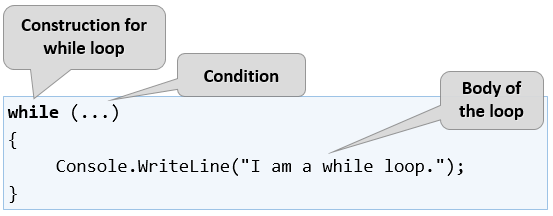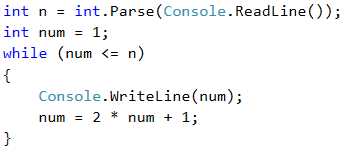# While Loop

The next type of loops that we will get familiar with are called while loops. The specific thing about them is that they repeat a block of commands while a condition is true. As a structure, they differ from for loops, and even have a simple syntax.

## Video: While Loop

Watch this video lesson to learn how to use the while-loop in C#: https://youtu.be/Jqnxl6k1V9w.

## While Loop – Explanation

In programming the while loop is used when we want to repeat the execution of a certain logic while a condition is in effect. By "condition," we understand every expression that returns true or false. When the condition is wrong, the while loop is interrupted, the program continues to execute the remaining code after the loop. The while loop construction looks like this:Here is a series of sample problems, the solution of which will help us better understand the use of the while loop.

## Example: Sequence of Numbers 2k+1

Write a program that prints all numbers ≤ n of the series: 1, 3, 7, 15, 31, …, assuming that each next number = previous number * 2 + 1.

Here is how we can solve the problem:

• We create a num variable for the current number to which we assign an initial value of 1.
• For a loop condition, we put the current number <= n.
• In the body of the loop: we print the value of the current number and increase the current number by using the formula from the problem's description.

Here is a sample implementation of this idea:### Testing in the Judge System

Test your solution here: https://judge.softuni.org/Contests/Practice/Index/514#4.